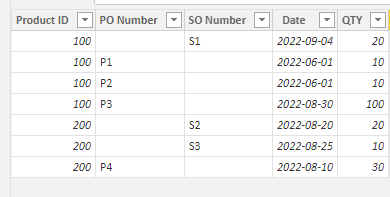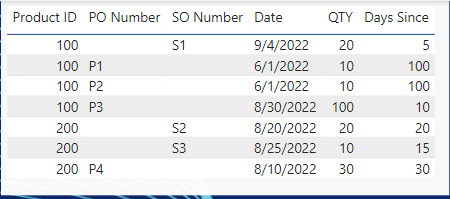cancel
Showing results for
Did you mean:

Fabric is Generally Available. Browse Fabric Presentations. Work towards your Fabric certification with the Cloud Skills Challenge.Frequent Visitor

## Avg Days on the Shelf

How could I calculate the avg days on the shelf from a table like this (rows with PO number is receiving, the ones with SO number are shipments)The way I approached this, I calculated the average days since received and subtracted the average days since shipped. The issue is when not all the received qty is shipped. If I have qty still sitting on the shelf, it skewes the average. The error increase with the ratio of onhand qty / shipped qty.

Thank you,

1 ACCEPTED SOLUTIONCommunity Support

Hi, @AyubSherif

According to your description, you want to judge whether all the things of a product have been shipped according to the [qty] field and. If the delivery is completed, the number of days between [date] and today () is taken; if the delivery is not completed, the number of days between the current [date] and the date value of the largest so number is taken. Right?

Here are the steps you can follow：

(1)This is my test data:(2)We can create a calculated column : “Days Since”

``````Days Since =
VAR _current_id = 'Table'[Product ID]
VAR _current_PO = 'Table'[PO Number]
VAR _current_SO = 'Table'[SO Number]
VAR _pro_PO =
SUMX (
FILTER (
'Table',
'Table'[Product ID] = _current_id
&& 'Table'[PO Number] <> BLANK ()
),
[QTY]
)
VAR _pro_SO =
SUMX (
FILTER (
'Table',
'Table'[Product ID] = _current_id
&& 'Table'[SO Number] <> BLANK ()
),
[QTY]
)
VAR _pro_max_SO =
MAXX (
FILTER (
'Table',
'Table'[Product ID] = _current_id
&& 'Table'[SO Number] <> BLANK ()
),
[Date]
)
RETURN
IF (
'Table'[PO Number] = BLANK (),
DATEDIFF ( 'Table'[Date], TODAY (), DAY ),
IF (
_pro_PO = _pro_SO,
DATEDIFF ( 'Table'[Date], TODAY (), DAY ),
DATEDIFF ( 'Table'[Date], _pro_max_SO, DAY )
)
)
``````

(3)Then we can meet your need , the result is as follow:If this method does not meet your needs, you can provide us with your special sample data and the desired output sample data in the form of tables, so that we can better help you solve the problem.

Best Regards,

Aniya Zhang

If this post helps, then please consider Accept it as the solution to help the other members find it more quickly

4 REPLIES 4Community Support

Hi, @AyubSherif

According to your description, you want to judge whether all the things of a product have been shipped according to the [qty] field and. If the delivery is completed, the number of days between [date] and today () is taken; if the delivery is not completed, the number of days between the current [date] and the date value of the largest so number is taken. Right?

Here are the steps you can follow：

(1)This is my test data:(2)We can create a calculated column : “Days Since”

``````Days Since =
VAR _current_id = 'Table'[Product ID]
VAR _current_PO = 'Table'[PO Number]
VAR _current_SO = 'Table'[SO Number]
VAR _pro_PO =
SUMX (
FILTER (
'Table',
'Table'[Product ID] = _current_id
&& 'Table'[PO Number] <> BLANK ()
),
[QTY]
)
VAR _pro_SO =
SUMX (
FILTER (
'Table',
'Table'[Product ID] = _current_id
&& 'Table'[SO Number] <> BLANK ()
),
[QTY]
)
VAR _pro_max_SO =
MAXX (
FILTER (
'Table',
'Table'[Product ID] = _current_id
&& 'Table'[SO Number] <> BLANK ()
),
[Date]
)
RETURN
IF (
'Table'[PO Number] = BLANK (),
DATEDIFF ( 'Table'[Date], TODAY (), DAY ),
IF (
_pro_PO = _pro_SO,
DATEDIFF ( 'Table'[Date], TODAY (), DAY ),
DATEDIFF ( 'Table'[Date], _pro_max_SO, DAY )
)
)
``````

(3)Then we can meet your need , the result is as follow:If this method does not meet your needs, you can provide us with your special sample data and the desired output sample data in the form of tables, so that we can better help you solve the problem.

Best Regards,

Aniya Zhang

If this post helps, then please consider Accept it as the solution to help the other members find it more quicklyFrequent VisitorFrequent Visitor

(1) What exactly is the "he avg days on the shelf" you want to calculate?

I am trying to calculate how long a product has stayed in our warehouse. The period from when it is received until it is shipped.

(2) The [Date] field indicates the date when the goods were received and sent, and the [QTY] field is the quantity of goods shipped. How is [Days since] calculated?

The days since is just Days since = DATEDIFF('Product History'[Date]TODAY(), DAY)

(3) How is "when not all the received qty is shipped/ qty still sitting on the shelf" judged?

Ex, when 100 items are received and only 20 shipped, I have 80 on the shelf (on hand).

For simplicity, take this table as an example:Here is my current measure:

Avg Days On Shelf =
VAR AVG_REC = CALCULATE(SUMX('Table','Table'[Days since]*'Table'[QTY]), 'Table'[PO number] <> BLANK())
VAR REC_QTY = CALCULATE(SUMX('Table','Table'[QTY]), 'Table'[PO number] <> BLANK())
VAR AVG_SH = CALCULATE(SUMX('Table','Table'[Days since]*'Table'[QTY]), 'Table'[SO number] <> BLANK())
VAR SH_QTY = CALCULATE(SUMX('Table','Table'[QTY]), 'Table'[SO number] <> BLANK())
RETURN
(AVG_REC/REC_QTY)-(AVG_SH/SH_QTY)
Here is my result:Intuitively, the answer for proudct "100" should be 95 days, but my unshipped qty is skeweing my receiving avg. The answer for product "200" is correct because everything shipped out. To summarize, this is a FIFO problem. How could I implement FIFO in dax to only account for products that have shipped out? Is there a mathimatical trick to better approximate the avg? (Basically to counter the onhand qty effect on my avg).Community Support

Hi, @AyubSherif

(1) What exactly is the "he avg days on the shelf" you want to calculate?

(2) The [Date] field indicates the date when the goods were received and sent, and the [QTY] field is the quantity of goods shipped. How is [Days since] calculated?

(3) How is "when not all the received qty is shipped/ qty still sitting on the shelf" judged?

(4) What data do you ultimately want the ball to have?

Can you show your sample data in the form of a table, and show us the results you want to get in the form of a table, so that we can better help you analyze the problem.

Best Regards,

Aniya Zhang

If this post helps, then please consider Accept it as the solution to help the other members find it more quickly.Announcements#### Power BI Monthly Update - November 2023

Check out the November 2023 Power BI update to learn about new features.#### Fabric Community News unified experience

Read the latest Fabric Community announcements, including updates on Power BI, Synapse, Data Factory and Data Activator.#### The largest Power BI and Fabric virtual conference

130+ sessions, 130+ speakers, Product managers, MVPs, and experts. All about Power BI and Fabric. Attend online or watch the recordings.Top Solution Authors
Top Kudoed Authors
Users online (3,476)